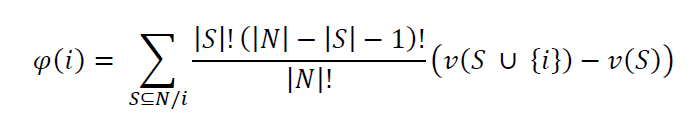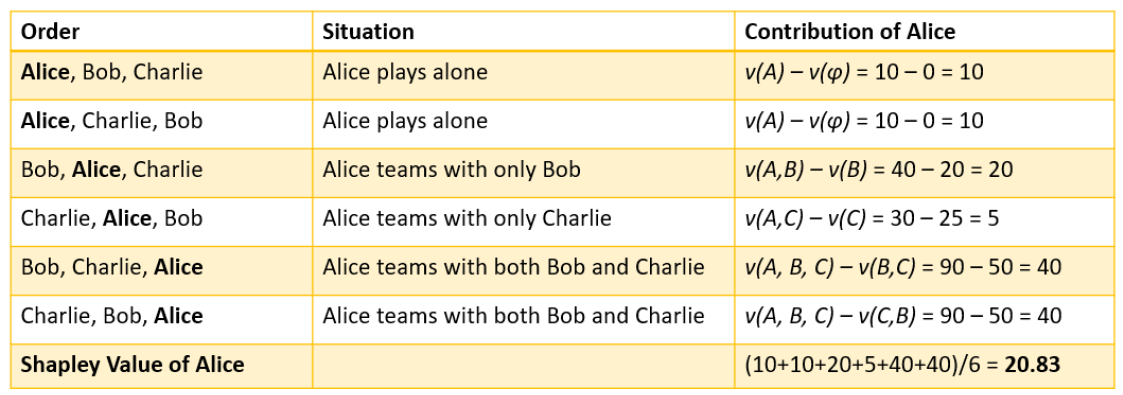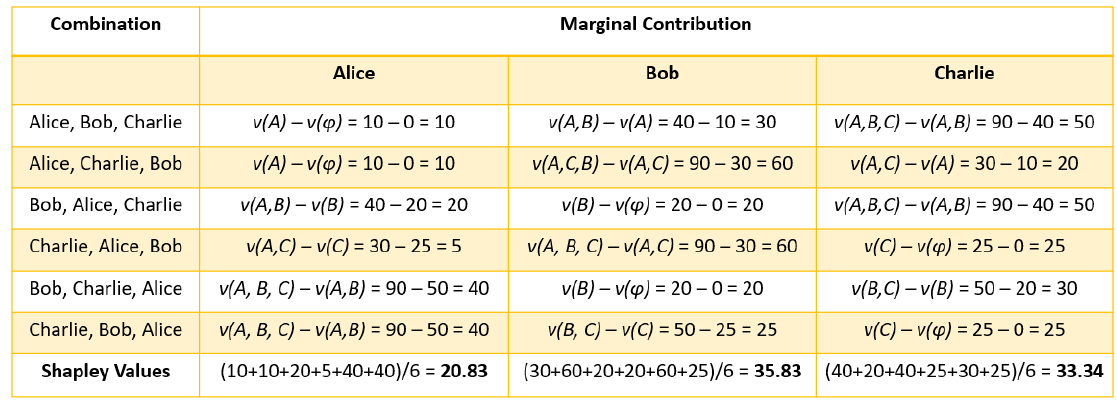# Understand the Workings of SHAP and Shapley Values Used in Explainable AI | by Aditya Bhattacharya | Aug, 2022

## Are you still confused about the working of SHAP and Shapley values? Let me provide the most simple and intuitive explanation of SHAP and Shapley values in this article.

SHapley Additive exPlanation (SHAP), which is another popular Explainable AI (XAI) framework that can provide model-agnostic local explainability for tabular, image, and text datasets.

SHAP is based on Shapley values, which is a concept popularly used in Game Theory. Although the mathematical understanding of Shapley values can be complicated, I will provide a simple, intuitive understanding of Shapley values and SHAP and focus more on the practical aspects of the framework.

The SHAP framework was introduced by Scott Lundberg and Su-In Lee in their research work, A Unified Approach of Interpreting Model Predictions. This was published in 2017. SHAP is based on the concept of Shapley values from cooperative game theory, it considers additive feature importance.

By definition, the Shapley value is the mean marginal contribution of each feature value across all possible values in the feature space. The mathematical understanding of Shapley values is complicated and might confuse most readers. That said, if you are interested in getting an in-depth mathematical understanding of Shapley values, we recommend that you take a look at the research paper called “A Value for n-Person Games.” Contributions to the Theory of Games 2.28 (1953), by Lloyd S. Shapley. In the next section, we will gain an intuitive understanding of Shapley values with a very simple example.

In this section, I will explain Shapley values using a very simple and easy-to-understand example. Let’s suppose that Alice, Bob, and Charlie are three friends who are taking part, as a team, in a Kaggle competition to solve a given problem with ML, for a certain cash prize. Their collective goal is to win the competition and get the prize money. All three of them are equally not good in all areas of ML and, therefore, have contributed in different ways. Now, if they win the competition and earn their prize money, how will they ensure a fair distribution of the prize money considering their individual contributions? How will they measure their individual contributions for the same goal? The answer to these questions can be given by Shapley values, which were introduced in 1951 by Lloyd Shapley.

The following diagram gives us a visual illustration of the scenario:

So, in this scenario, Alice, Bob, and Charlie are part of the same team, playing the same game (which is the Kaggle competition). In game theory, this is referred to as a Coalition Game. The prize money for the competition is their payout. So, Shapley values tell us the average contribution of each player to the payout ensuring a fair distribution. But why not just equally distribute the prize money between all the players? Well, since the contributions are not equal, it is not fair to distribute the money equally.

Now, how do we decide the fairest way to distribute the payout? One way is to assume that Alice, Bob, and Charlie joined the game in a sequence in which Alice started first, followed by Bob, and then followed by Charlie. Let’s suppose that if Alice, Bob, and Charlie had participated alone, they would have gained 10 points, 20 points, and 25 points, respectively. But if only Alice and Bob teamed up, they might have received 40 points. While Alice and Charlie together could get 30 points, Bob and Charlie together could get 50 points. When all three of them collaborate together, only then do they get 90 points, which is sufficient for them to win the competition.This table illustrates the point values for each condition for calculating the average marginal contribution of each player

Mathematically, if we assume that there are N players, where S is the coalition subset of players and 𝑣(𝑆) is the total value of S players, then by the formula of Shapley values, the marginal contribution of player i is given as follows:Equation for Marginal contribution of player i

The equation of Shapley value might look complicated, but let’s simplify this with our example. Please note that the order in which each player starts the game is important to consider as Shapley values try to account for the order of each player to calculate the marginal contribution.

Now, for our example, the contribution of Alice can be calculated by calculating the difference that Alice can cause to the final score. So, the contribution is calculated by taking the difference in the points scored when Alice is in the game and when she is not.

Also, when Alice is playing, she can either play alone or team up with others. When Alice is playing, the value that she can create can be represented as 𝑣(𝐴). Likewise, 𝑣(𝐵) and 𝑣(𝐶) denote individual values created by Bob and Charlie. Now, when Alice and Bob are teaming up, we can calculate only Alice’s contribution by removing Bob’s contribution from the overall contribution. This can be represented as 𝑣(𝐴, 𝐵)– 𝑣(𝐵) . And if all three are playing together, Alice’s contribution is given as 𝑣(𝐴, 𝐵, 𝐶)– 𝑣(𝐵, 𝐶).

Considering all possible permutations of the sequences by which Alice, Bob, and Charlie play the game, the marginal contribution of Alice is the average of her individual contributions in all possible scenarios.The Shapley value of Alice is her marginal contribution considering all possible scenarios

So, the overall contribution of Alice will be her marginal contribution across all possible scenarios, which also happens to be the Shapley value. For Alice, the Shapley value is 20.83. Similarly, we can calculate the marginal contribution for Bob and Charlie, as shown in the following table:Marginal contribution for Alice, Bob, and Charlie

I hope this wasn’t too difficult to understand! One thing to note is that the sum of marginal contributions of Alice, Bob, and Charlie should be equal to the total contribution made by all three of them together. Now, let’s try to understand Shapley values in the context of ML.

In order to understand the importance of Shapley values in ML to explain model predictions, we will try to modify the example about Alice, Bob, and Charlie that we used for understanding Shapley values. We can consider Alice, Bob, and Charlie to be three different features present in a dataset used for training a model. So, in this case, the player contributions will be the contribution of each feature. The game or the Kaggle competition will be the black-box ML model and the payout will be the prediction. So, if we want to know the contribution of each feature toward the model prediction, we will use Shapley values.Understanding Shapley values in the context of ML (Image by Author)

Therefore, Shapley values help us to understand the collective contribution of each feature toward the outcome predicted by black-box ML models. By using Shapley values, we can explain the working of black-box models by estimating the feature contributions.

In this article, we focused on understanding the importance of the SHAP framework for model explainability. By now, you have a good understanding of Shapley values and SHAP.

1. GitHub repo for Python SHAP framework — https://github.com/slundberg/shap
2. Applied Machine Learning Explainability Techniques — https://amzn.to/3cY4c2h